Time left:

#### SSC CHSL Date: 13_04_2021 Shift: S2

 Q.1 In a certain code language, ROCKET is written as BNQSDJ. How will NOTICE be written in that language?
 1. SNMDBH
 2. UPOFDJ
 3. SPMFBJ
 4. NMHSDB
 Q.2 Which two signs should be interchanged to make the following equation correct? 14 + 12 × 18 ÷ 6 – 12 = 6
 1. × and +
 2. ÷ and ×
 3. ÷ and −
 4. − and +
 Q.3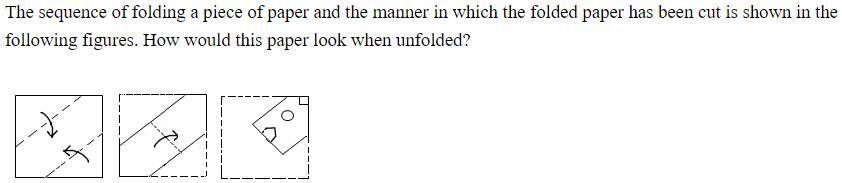1.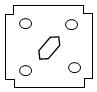2.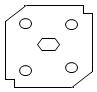3.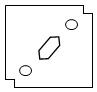4.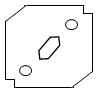Q.4 Select the option that is related to the third letter-cluster in the same way as the second letter-cluster is related to the first letter-cluster. MORV : NJGE : : DGKP : ?
 1. ZVSQ
 2. PLIG
 3. WRNK
 4. WTPK
 Q.5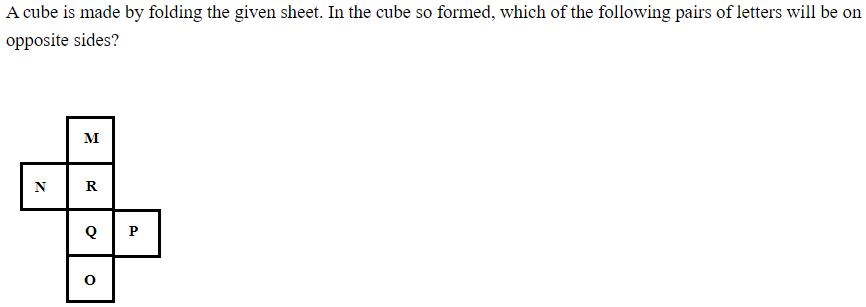1.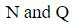2.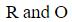3.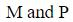4.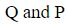Q.6 Four numbers have been given, out of which three are alike in some manner and one is different. Select the number that is different.
 1. 4095
 2. 255
 3. 1049
 4. 127
 Q.7 Select the option in which the numbers are related in the same way as are the numbers of the following set. {22, 17, 31}
 1. {14, 8, 23}
 2. {16, 9, 25}
 3. {18, 23, 27}
 4. {31, 26, 40}
 Q.8 Select the option in which the words share the same relationship as that shared by the given pair of words.Pullover : Wool
 1. Prism : Glass
 2. Fabric : Ore
 3. Linen : Latex
 4. Pulp : Sack
 Q.9 Select the combination of letters that when sequentially placed in the blanks of the given series will complete the series. K O _ M C L _ Q M C M O _ M _ N O Q _ C
 1. M, O, Q, C, O
 2. M, O, Q, C, M
 3. Q, O, C, Q, M
 4. Q, O, Q, C, M
 Q.10 Four words have been given, out of which three are alike in some manner and one is different. Select the word that is different.
 1. Director
 2. Minister
 3. Speaker
 4. Mayor
 Q.11 ‘A + B’ means ‘A is the wife of B’. ‘A – B’ means ‘A is the daughter of B’. ‘A × B’ means ‘A is the sister of B’. ‘A ÷ B’ means ‘A is the brother of B’. If P ÷ M × R – Z + Q, then how is P related to Q?
 1. Son
 2. Son-in-law
 3. Nephew
 4. Brother
 Q.12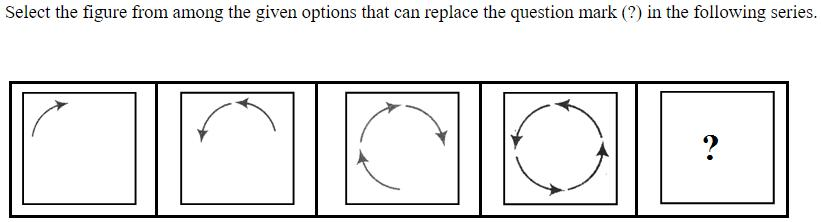1.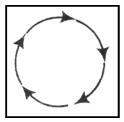2.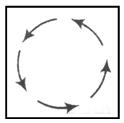3.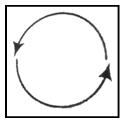4.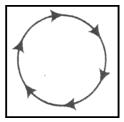Q.13 Four letter-clusters have been given, out of which three are alike in some manner and one is different. Select the letter-cluster that is different.
 1. HDFZ
 2. FBDX
 3. TPRL
 4. SNPJ
 Q.14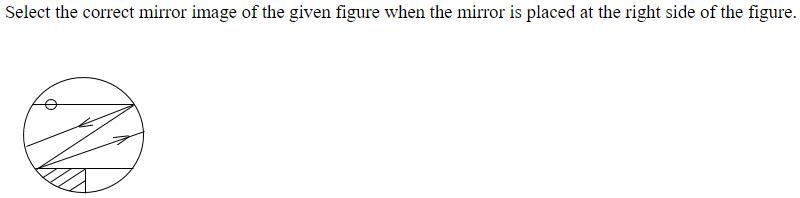1.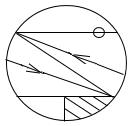2.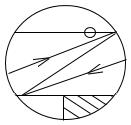3.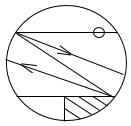4.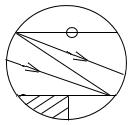Q.15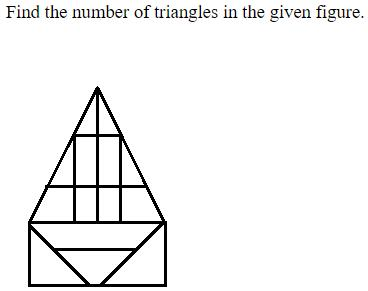1.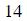2.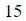3.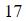4.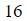Q.16 In a certain code language, REFUSAL is coded as 100 and SHORTEN is coded as 83. How will INERTIA be coded in that language?
 1. 150
 2. 105
 3. 106
 4. 160
 Q.17 Select the option that is related to the third number in the same way as the second number is related to the first number. 256 : 13 : : 676 : ?
 1. 24
 2. 23
 3. 26
 4. 25
 Q.18 Select the number from among the given options that can replace the question mark (?) in the following series. 1, 2, 6, 21, ?, 445
 1. 44
 2. 94
 3. 66
 4. 88
 Q.19 Select the correct option that indicates the arrangement of the given words in the order in which they appear in an English dictionary. 1. Certificate 2. Cerebellum 3. Cervical 4. Ceremonious 5. Certainty
 1. 2, 5, 4, 1, 3
 2. 2, 4, 5, 1, 3
 3. 2, 5, 1, 3, 4
 4. 2, 3, 1, 5, 4
 Q.20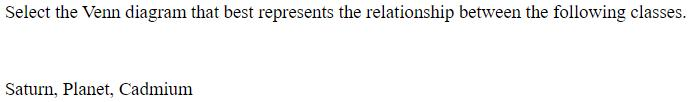1.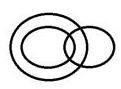2.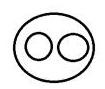3.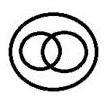4.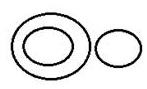Q.21 ‘War’ is related to ‘Destruction’ in the same way as ‘Cyclone’ is related to ‘______’.
 1. Wind
 2. Storm
 3. Hurricane
 4. Devastation
 Q.22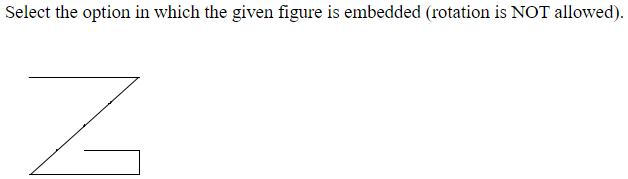1.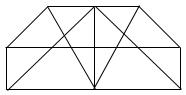2.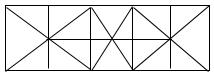3.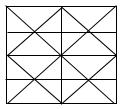4.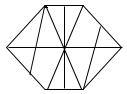Q.23 Read the given statements and conclusions carefully. Assuming that the information given in the statements is true, even if it appears to be at variance with commonly known facts, decide which of the given conclusions logically follow(s) from the statements.Statements:Some men are athletes.No athlete is a singer.All singers are dancers.Conclusions:I. No dancer is an athlete.II. Some singers are men.III. Some men are dancers.
 1. None of the conclusions follow .
 2. Only conclusion I follows.
 3. Only conclusions II and III follow .
 4. Only conclusion II follows .
 Q.24 From the given options choose the one in which the set of numbers do not follow the same Logic/Rule/Relation as the numbers given in below set.(12, 36, 132)
 1. (22, 66, 242)
 2. (18, 54, 196)
 3. (19, 57, 209)
 4. (15, 45, 165)
 Q.25 An online entrance exam was conducted in 250 centres all over a country. The average number of applicants per centre was found to be 1250. However, it was later realised that in one centre, the number of applicants was counted as 1758 instead of 1658. What was the correct average number of applicants per centre?
 1. 1429.6
 2. 1294.6
 3. 1249.6
 4. 1492.6
Questions
1
2
3
4
5
6
7
8
9
10
11
12
13
14
15
16
17
18
19
20
21
22
23
24
25